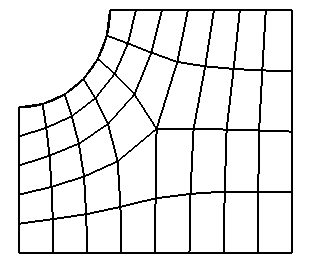# Pentagon

Applies to: Surfaces

Summary: Produces a pentagon-primitive mesh for a surface

Syntax:

Surface <range> Scheme Pentagon

Discussion:

The pentagon scheme is a meshing primitive for 5-sided regions. It is similar to the triprimitive and polyhedron schemes, but is hard-coded for 5 sided surfaces.

The pentagon scheme indicates the region should be meshed as a pentagon. The scheme works best if the shape has 5 well-defined corners; however shapes with more corners can be meshed. The algorithm requires that there be at least 10 intervals (2 per side) specified on the curves representing the perimeter of the surface. In addition, the sum of the intervals on any three connected sides must be at least two greater than the sum of the intervals on the remaining two sides. Figure 1 shows two examples of pentagon meshes.Figure 1. Examples of Pentagon Scheme Meshes